CLAT  >  Simple Interest and Compound Interest

# Simple Interest and Compound Interest - Quantitative Techniques for CLAT

 Table of contentsSimple Interest and Compound InterestSimple InterestCompound InterestSolved Examples## Simple Interest and Compound Interest

Principal is the amount on which interest is paid / earned.

If principal is denoted by P

The rate of interest is denoted by R

The time for which the principal is given on interest = T

Then

### ## Simple Interest

Simple interest earned / paid is devoted by S.I.

Then (1) S.I. =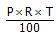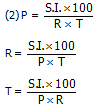Amount (A) = P + SI (Amount is the sum of P and the SI earned)

Amount x 100 = Principal x (100 + RT)

Principal =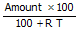## Compound Interest

Amount (A) =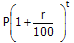Or CI = A – P

Difference of CI and SI for a period of one year in zero

For 2 years the difference of CI and SI is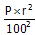For 3 years the difference of CI and SI is

=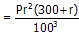## Solved Examples

Example 1: Ramesh deposited Rs. 29400 for 6 years at simple interest. After 6 years he received Rs. 4200 as interest. Find the annual rate of interest.

• P = Rs. 29400             T = 6 years        SI = Rs. 4200

For finding out the rate of interest R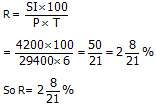Example 2: I invested an amount of Rs. 17500 at the rate of 8% per annum. After how many years will I get Rs. 16800 as simple interest?

• P = Rs. 17500            R = 8%             SI = Rs.16800

So T =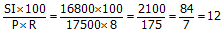So time is 12 years

Example 3: Pritam invested Rs. 16840 at 6% for 5 years. What amount of SI, he will get after 5 years.

• P = 16840

R = 6%

T = 5 years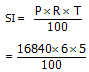= RS. 5052

Example 4: At what rate percent per annum simple interest a sum of money doubles itself in 15 years.

• let the principal be Rs. 100

T = 15 years

SI = Rs.100

R = ?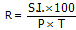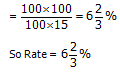Example 5: A sum of Rs. 3100 was lent partly at 5% and partly at 8% simple interest. The total interest received after 3 years was Rs. 600. Amount lent on 5% per annum was how much.

• let Rs. X be lent at 5%, then the remaining amount of Rs. (3100-x) was lent at 8%

So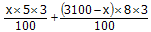Or 15x + 24 (3100 – x) = 60000

Or 9x = 74400 – 60000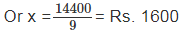### Short Cut Method

Let us suppose that whole amount was given at 5%

Interest =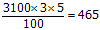But the interest earned in 600. It means some amount which earns Rs. 135 at 3% interest (8% - 5%) was given. This amount is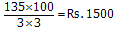So 3100 – 1500 = Rs. 1600 was given an interest of 5%.

Example 6: The simple interest on a sum of money is 4/9 of the principal and the number of years is equal to the rate percent per annum. The rate per annum is what?

• Let the amount (Principal) be Rs. 1

SI = Rs. 4/9

Let rate be = x%

Then T = x years

As per the formula SI = PRT / 100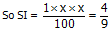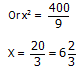So rate per annum =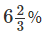Example 7: In 4 years, the simple interest on a sum of money is 9/25 of the principal. Find out the annual rate of interest.

• Let the principal be Rs. 1

Interest =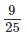T = 4 years

R =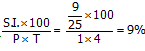Example 8: A sum of money at simple interest amounts to Rs. 1012 in 2½ years and to Rs. 1067.20 in 4 years. Find out the rate of interest, per year.

Solution: From the question it is clear that

Rs. 1067.20 – Rs. 1012.00 = Rs. 55.20 is the simple interest earned in a period of 1½ years. So now we can find the interest earned in 2½ year which will be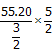= 18.40 x 5 = 92.00

So the principal in the beginning was Rs. 1012-92 = Rs. 920

So now P = Rs. 920

T = 5/2 year

Simple Interest = Rs. 92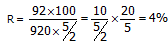## Compound Interest

To understand the method of calculating compound interest, let us consider an example.

Example: I borrowed Rs. 40,000 from a bank. The bank charges interest at the rate of 10% per annum. At the end of the year I will have to pay Rs.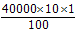i.e. Rs. 4000 as interest.

Thus total amount of Rs. 40000 + Rs. 4000 = Rs. 44000 will have to be paid back to the bank.

If due to some reason I am not able to pay, the bank will charge 10% interest for the next year on the principal of Rs. 44000. At the end of the 2nd year the interest will be Rs.= Rs. 4400.

Thus total interest payable to the bank will be Rs. (4400 + 4000) = Rs. 8400. This interest is Rs. 400 more than the simple interest on 40000 for 2 years at 10%. This Rs. 400 is the interest on the interest of 4000 for the first year. So the interest calculated in this way is called compound interest.

Remarks: For the first unit of time the simple and the compound interest are equal. From the second unit of time onwards the compound interest is more than the simple interest.

Example 1: find the compound interest on Rs. 3000 for 3 years at 8% per annum.

• Principal for the first year = Rs. 3000

Rate = 8%

Time = one year

Interest =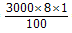= Rs. 240

Amount = Principal + interest = Rs. 3000 + 240 = Rs. 3240

Principal for 2nd year = Rs. 3240

Rate = 8%

Time = 1 year

So interest for 2nd year =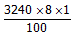So amount = Rs. 3240 + Rs. 259.20 = Rs. 3499.20

Principal for 3rd year = Rs. 3499.20

Rate = 8%

Time = 1 year

Interest for 3rd year =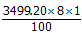So compound interest for 3 years

= Rs. 240 + Rs. 259.20 + Rs. 279.94 = Rs. 779.14

When interest is compounded half yearly.

Example 2: Find the compound interest on Rs. 1000 for 1½ year at 12% per annum when the interest is compounded half yearly.

• Rate per annum is 12%

So the rate per half year will be 12/2 % or 6%. Also there will be 3 half years in 1½ year. By using the formula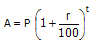In this case P = 1000

r = 6% per half year

t = 3 half years

So A =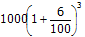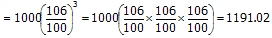So compound interest = Rs. 1191.02 – Rs. 1000 = Rs. 191.02

When the interest is compounded quarterly

Example 3: Find the compound interest on Rs. 6000 for one year at 16% per annum when the interest is compounded quarterly.

• Rate per annum is 16%, so rate per quarter is 16/4 = 4%

Time is one year or 4 quarters

By the formula of compound interest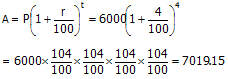So compound interest for 4 quarters at the rate of 16% on 6000 is 7019.15 – 6000 = Rs. 1019.15

Example 4: At what rate per cent interest per annum, will Rs. 10000 amount to Rs. 14641 in 2 years. If the interest is compounded half yearly.

• Here P = Rs. 10000

A = Rs. 14641

R = ?

T = 2 years or 4 half years

Formula isA =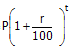or 14641 =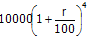or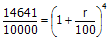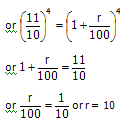so 10% is half yearly rate

annual rate will be 10 x 2 = 20%

Example 5: The difference between the compound interest and simple interest on a certain sum at 14% per annum for 2 years is Rs. 147. Find the sum.

• Let the sum be Rs. 100

SI on 100 for 2 years at 14% is =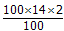CI on 100 for 2 years at 14% = A – P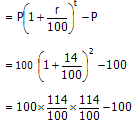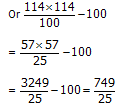Difference in CI and SI =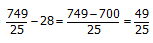If difference is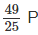= 100

If difference 1=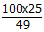If difference is Rs. 147 =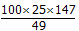The document Simple Interest and Compound Interest | Quantitative Techniques for CLAT is a part of the CLAT Course Quantitative Techniques for CLAT.
All you need of CLAT at this link: CLAT

## Quantitative Techniques for CLAT

46 videos|69 docs|95 tests

## FAQs on Simple Interest and Compound Interest - Quantitative Techniques for CLAT

 1. What is simple interest?Ans. Simple interest is a type of interest that is calculated only on the principal amount of a loan or investment. It does not take into account any accumulated interest over time. The formula for calculating simple interest is: Interest = (Principal amount x Interest rate x Time period).
 2. What is compound interest?Ans. Compound interest is a type of interest that is calculated on both the principal amount and any accumulated interest from previous periods. This means that the interest amount keeps increasing as time goes on. The formula for calculating compound interest is: A = P(1 + r/n)^(nt), where A is the final amount, P is the principal amount, r is the annual interest rate, n is the number of times that interest is compounded per year, and t is the number of years.
 3. How does simple interest differ from compound interest?Ans. Simple interest is calculated only on the principal amount, while compound interest takes into account both the principal amount and any accumulated interest. This means that compound interest will generally result in a higher total amount compared to simple interest over the same time period.
 4. What are the advantages of simple interest?Ans. Simple interest is easier to calculate and understand compared to compound interest. It is often used for short-term loans or investments where the interest accumulated over time is not significant. Simple interest is also beneficial for borrowers, as the total amount owed remains constant throughout the loan term.
 5. What are the advantages of compound interest?Ans. Compound interest allows for exponential growth of investments over time. It is particularly advantageous for long-term investments or savings accounts, as the interest compounds and increases the overall return. Compound interest can help individuals build wealth and achieve financial goals.

## Quantitative Techniques for CLAT

46 videos|69 docs|95 testsExplore Courses for CLAT examSignup to see your scores go up within 7 days! Learn & Practice with 1000+ FREE Notes, Videos & Tests.
10M+ students study on EduRev
Track your progress, build streaks, highlight & save important lessons and more!
Related Searches

,

,

,

,

,

,

,

,

,

,

,

,

,

,

,

,

,

,

,

,

,

;